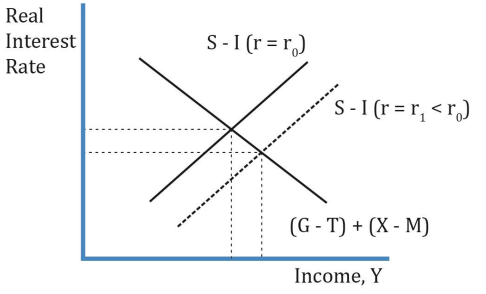# I don't know where the hell is the IS curve in this graph

Hi guys,
So IS curve represents the points where aggregate expenditure = aggregate income.
C + S + T (Aggregate income) = C + I + G + (X - M) (Aggregate expenditure). All good so far.
But for whatever reason, when we want to plot it (the IS curve), we tweak it to make it look like this:
S - I = C + I + G + (X - M). With this expression we aren’t even equating strictly Aggregate income with Aggregate expenditure, but we started from there to come to that expression, so i guess it’s mathematically “right”.

Then, here is the result of the plot, and this graph just states “The graph below shows an IS curve”:Excuse my ignorance, but all i’m seeing is an “S - I” curve and an “(G - T) + (X - M)” curve, no IS curve. Where the hell is it?

There isn’t an IS curve in that graph; that’s why you don’t see it.

The label on the vertical axis is wrong: it isn’t real interest rate; it’s S − I or (G − T) + (X − M); i.e., it’s money.

To get the IS curve, you have to plot the equilibrium income values (where the (G − T) + (X − M) curve intersects each S − I curve) on the horizontal axis against the real interest rate (r0, r1, and so on) on the vertical axis.

1 Like

Thanks S2000magician but i’m not sure i understood this part:

The label on the vertical axis is wrong: it isn’t real interest rate; it’s S − I or (G − T) + (X − M); i.e., it’s money.

S - I = Money // (G - T) + (X - M) = Money? I’ve never heard of that!

The IS name is a notional name for Loanable Funds Market. Where ‘S National’ = I. And, or, (y-c(y-t)-g)=I(r).

The above graph represents the dichotomy of either side of the IS equation before it is calculated along side the LM (Money Market) equation.